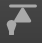﻿ MechDesigner Reference & User Interface > 2.2 Mechanism-Editor: > Force elements > Forces | Show Force Vectors

# Show/Hide Force Vectors

Forces toolbar > Show Force VectorsVisibility toolbar > Show Force-Vectors - is activeVisibility toolbar > Hide Force-Vectors - is not active Menu : Add menu > Forces sub-menu > Display Force Vectors Force Vectors show only when... •Force toolbar > Calculate Forces is active •A minimum of one kinematic-chain is kinematically-defined •A minimum of one Part in the kinematic-chain has mass(kg)- see Cad-Line dialog-box | Mass Properties •Filters toolbar > Display Part-Outlines is active Force-Vectors in the graphic-area •Location: radiate from Pin-Joints and Slide-Joints, Springs, Cam Contacts, gear contacts, belts. •Color: each Force Vector that acts on a Part is the color of the Part-Outline (see below - colors: Part-Outlines and Force-Vectors). •Direction: direction of the force •Length: scaled to the magnitude (see also: Vector scaling buttons). •Magnitude: at the arrowhead of each vector Rotary Motor Moment / Torque •Moment Location: at each Pin-Joint with a Rotary Motor (see also: Configure Power Source) •Moment Length: proportional to the magnitude of the moment/torque Linear Motor Driving Force •Vector Location: at each Slide-Joint with a Linear Motor (see also: Configure Power Source) •Vector Length: proportional to the magnitude of the driving force to balance the Load. Colors: Part-Outlines and Force-Vectors When you show Force-Vectors, each Part-Outline becomes the same color as the Force-Vectors that ACT-ON the Part. To identify the Force-Vectors that act on a Part: hover above a Part-Outline; the Part-Outline AND the Force-Vectors will become RED.   Use the Configure Power Source to edit the color of each Force-Vector, or hide the Force-Vector. See also: Kinetostatic Torque and Speed dialog